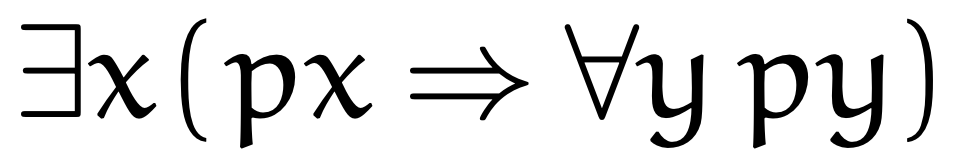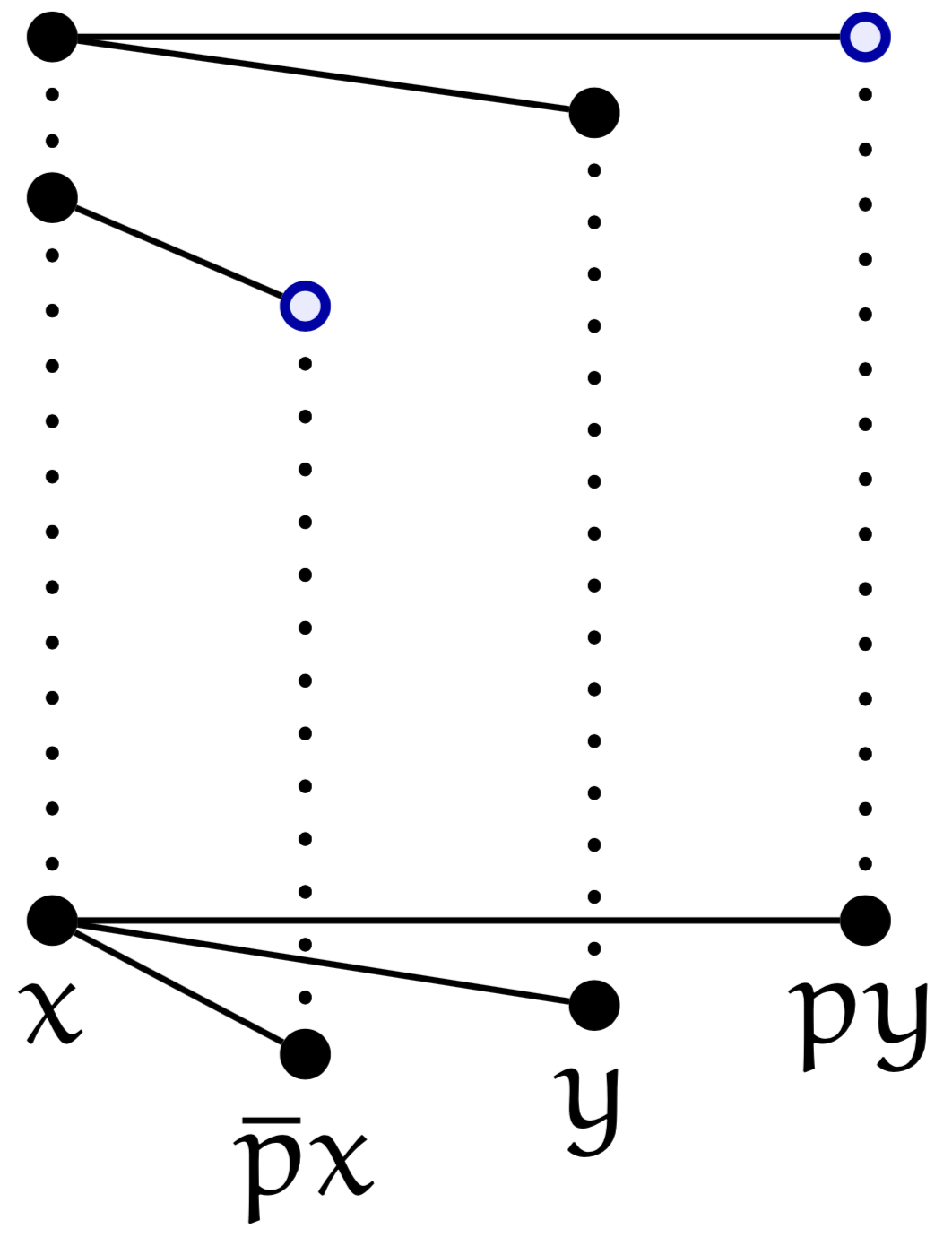### Dominic Hughes - Selected Papers

First-order proofs without syntax [pdf - bibtex]
arxiv.org:1906.11236, June 2019

Abstract
Proofs are traditionally syntactic, inductively generated objects. This paper reformulates first-order logic (predicate calculus) with proofs which are graph-theoretic rather than syntactic. It defines a combinatorial proof of a formula ϕ as a lax fibration over a graph associated with ϕ. The main theorem is soundness and completeness: a formula is a valid if and only if it has a combinatorial proof.

Example
A combinatorial proof ofis shown below.